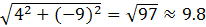### Sample Problem

Given that the vector v has an initial point at (1, -2) and a terminal point at (5, -11),

a)What is the component form of v?

< , >

b)What is the magnitude of vector v? Round your answer to one decimal point.

#### Solution

a)The component form of v=<5-1, -11-(-2)>=<4, -9>

b)The magnitude is ||v||=